# ImageJ with Python Kernel¶

This notebook covers how to use ImageJ as a library from Python. A major advantage of this approach is the ability to combine ImageJ with other tools available from the Python software ecosystem, including NumPy, SciPy, scikit-image, CellProfiler, OpenCV, ITK and more.

This notebook assumes familiarity with the ImageJ API. Detailed tutorials in that regard can be found in the other notebooks.

## Starting ImageJ from Python¶

The pyimagej module enables access to the entire ImageJ API from Python in a natural way.

Let's initialize an ImageJ gateway including Fiji plugins, at a reproducible version:

In :
import imagej
ij = imagej.init('sc.fiji:fiji:2.0.0-pre-10')
ij.getVersion()

Out:
'2.0.0-rc-71/1.52i'

### Ways to initialize¶

Requirement Code1 Reproducible?2
Newest available version of ImageJ ij = imagej.init() NO
Specific version of ImageJ ij = imagej.init('net.imagej:imagej:2.0.0-rc-71') YES
With a GUI (newest version) ij = imagej.init(headless=False) NO
With a GUI (specific version) ij = imagej.init('net.imagej:imageJ:2.0.0-rc-71', headless=False) YES
With support for ImageJ 1.x (newest versions) ij = imagej.init('net.imagej:imagej+net.imagej:imagej-legacy') NO
With Fiji plugins (newest version) ij = imagej.init('sc.fiji:fiji') NO
With Fiji plugins (specific version) ij = imagej.init('sc.fiji:fiji:2.0.0-pre-10') YES
From a local installation ij = imagej.init('/Applications/Fiji.app') DEPENDS

1 pyimagej uses jgo internally to call up ImageJ, so all of these initializations are tied to the usage of jgo. You can read up on the usage of jgo to find out more about this initialization.

2 Reproducible means code is stable, executing the same today, tomorrow, and in years to come. While it is convenient and elegant to depend on the newest version of a program, behavior may change when new versions are released—for the better if bugs are fixed; for the worse if bugs are introduced—and people executing your notebook at a later time may encounter broken cells, unexpected results, or other more subtle behavioral differences. You can help avoid this pitfall by pinning to a specific version of the software. The British Ecological Society published Guide to Better Science: Reproducible Code diving into the relevant challenges in more detail, including an R-centric illustration of best practices. A web search for reproducible python also yields several detailed articles.

### Increasing the memory available to Java¶

Java's virtual machine (the JVM) has a "max heap" value limiting how much memory it can use. You can increase it:

import scyjava_config
import imagej
ij = imagej.init()


Replace 6g with the amount of memory Java should have. You can also pass other JVM arguments.

Without having specified the max heap value explicitly, here is how much memory this notebook's JVM has available:

In :
ij.getApp().getInfo(True)

Out:
'ImageJ 2.0.0-rc-71/1.52i; Java 1.8.0_192 [x86_64]; 228MB of 3641MB'

## A simple example: ij.py.show()¶

ImageJ can display numpy images using ij.py.show. Let's demonstrate using scikit-image to grab a sample:

In :
from skimage import io
import numpy as np
img = np.mean(img[500:1000,300:850], axis=2)
ij.py.show(img, cmap = 'gray')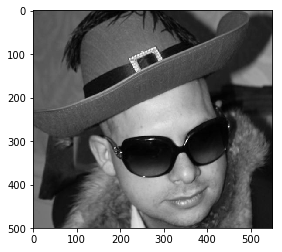## Converting to Java: ij.py.to_java¶

The function to_java is capable of converting common Python and numpy data types into their Java/ImageJ equivalent. There is one important nuance; converting a numpy array to java creates a java object that points to the numpy array. This means that changing the java object also changes the numpy array.

Let's take a look at lists:

In :
# Lists convert and handle simply
ex_list = [1, 2, 3, 4]
print(type(ex_list))
java_list = ij.py.to_java(ex_list)
print(type(java_list))

<class 'list'>
<class 'jnius.reflect.java.util.ArrayList'>


A java list can be accessed the same as a python list. Changing values in the python list does not change values in the java_list

In :
ex_list = 4
java_list

Out:
1

By contrast, ops can operate on numpy arrays and change them, though you need to wrap the arrays in to_java first.

In :
import numpy as np
arr1 = np.array([[1, 2], [3, 4]])
arr2 = np.array([[5, 6], [7, 8]])
arr_output = ij.py.new_numpy_image(arr1)

ij.op().run('multiply', ij.py.to_java(arr_output), ij.py.to_java(arr1), ij.py.to_java(arr2))
arr_output

Out:
array([[ 5., 12.],
[21., 32.]])

### Technical note for using ops on numpy arrays¶

Numpy arrays become RandomAccessibleIntervals and can substitute for IterableIntervals.

In :
print(type(ij.py.to_java(arr1)))

<class 'jnius.reflect.net/imglib2/python/ReferenceGuardingRandomAccessibleInterval'>


If you need to trouble shoot op workings, look for implementations that use only IterableIntervals or RandomAccessibleIntervals. To find the implementations use the print(ij.op().help()) function.

For the multiply function the implementation we used is second to last (net.imagej.ops.math.IIToRAIOutputII$Multiply) In : # Print is required to render newlines print(ij.op().help('multiply'))  Available operations: (ArrayImg arg) = net.imagej.ops.math.ConstantToArrayImageP$MultiplyByte(
ArrayImg arg,
byte value)
(ArrayImg arg) =
net.imagej.ops.math.ConstantToArrayImageP$MultiplyDouble( ArrayImg arg, double value) (ArrayImg arg) = net.imagej.ops.math.ConstantToArrayImageP$MultiplyFloat(
ArrayImg arg,
float value)
(ArrayImg arg) =
net.imagej.ops.math.ConstantToArrayImageP$MultiplyInt( ArrayImg arg, int value) (ArrayImg arg) = net.imagej.ops.math.ConstantToArrayImageP$MultiplyLong(
ArrayImg arg,
long value)
(ArrayImg arg) =
net.imagej.ops.math.ConstantToArrayImageP$MultiplyShort( ArrayImg arg, short value) (ArrayImg arg) = net.imagej.ops.math.ConstantToArrayImageP$MultiplyUnsignedByte(
ArrayImg arg,
byte value)
(ArrayImg arg) =
net.imagej.ops.math.ConstantToArrayImageP$MultiplyUnsignedInt( ArrayImg arg, int value) (ArrayImg arg) = net.imagej.ops.math.ConstantToArrayImageP$MultiplyUnsignedLong(
ArrayImg arg,
long value)
(ArrayImg arg) =
net.imagej.ops.math.ConstantToArrayImageP$MultiplyUnsignedShort( ArrayImg arg, short value) (ArrayImg arg) = net.imagej.ops.math.ConstantToArrayImage$MultiplyByte(
ArrayImg arg,
byte value)
(ArrayImg arg) =
net.imagej.ops.math.ConstantToArrayImage$MultiplyDouble( ArrayImg arg, double value) (ArrayImg arg) = net.imagej.ops.math.ConstantToArrayImage$MultiplyFloat(
ArrayImg arg,
float value)
(ArrayImg arg) =
net.imagej.ops.math.ConstantToArrayImage$MultiplyInt( ArrayImg arg, int value) (ArrayImg arg) = net.imagej.ops.math.ConstantToArrayImage$MultiplyLong(
ArrayImg arg,
long value)
(ArrayImg arg) =
net.imagej.ops.math.ConstantToArrayImage$MultiplyShort( ArrayImg arg, short value) (ArrayImg arg) = net.imagej.ops.math.ConstantToArrayImage$MultiplyUnsignedByte(
ArrayImg arg,
byte value)
(ArrayImg arg) =
net.imagej.ops.math.ConstantToArrayImage$MultiplyUnsignedInt( ArrayImg arg, int value) (ArrayImg arg) = net.imagej.ops.math.ConstantToArrayImage$MultiplyUnsignedLong(
ArrayImg arg,
long value)
(ArrayImg arg) =
net.imagej.ops.math.ConstantToArrayImage$MultiplyUnsignedShort( ArrayImg arg, short value) (IterableInterval out?) = net.imagej.ops.math.IIToIIOutputII$Multiply(
IterableInterval out?,
IterableInterval in1,
IterableInterval in2)
(NumericType out?) =
net.imagej.ops.math.NumericTypeBinaryMath$Multiply( NumericType out?, NumericType in1, NumericType in2) (int result) = net.imagej.ops.math.PrimitiveMath$IntegerMultiply(
int a,
int b)
(long result) =
net.imagej.ops.math.PrimitiveMath$LongMultiply( long a, long b) (float result) = net.imagej.ops.math.PrimitiveMath$FloatMultiply(
float a,
float b)
(double result) =
net.imagej.ops.math.PrimitiveMath$DoubleMultiply( double a, double b) (RealType out) = net.imagej.ops.math.BinaryRealTypeMath$Multiply(
RealType out,
RealType in1,
RealType in2)
(IterableInterval out?) =
net.imagej.ops.math.ConstantToIIOutputII$Multiply( IterableInterval out?, IterableInterval in, NumericType value) (PlanarImg arg) = net.imagej.ops.math.ConstantToPlanarImage$MultiplyByte(
PlanarImg arg,
byte value)
(PlanarImg arg) =
net.imagej.ops.math.ConstantToPlanarImage$MultiplyDouble( PlanarImg arg, double value) (PlanarImg arg) = net.imagej.ops.math.ConstantToPlanarImage$MultiplyFloat(
PlanarImg arg,
float value)
(PlanarImg arg) =
net.imagej.ops.math.ConstantToPlanarImage$MultiplyInt( PlanarImg arg, int value) (PlanarImg arg) = net.imagej.ops.math.ConstantToPlanarImage$MultiplyLong(
PlanarImg arg,
long value)
(PlanarImg arg) =
net.imagej.ops.math.ConstantToPlanarImage$MultiplyShort( PlanarImg arg, short value) (PlanarImg arg) = net.imagej.ops.math.ConstantToPlanarImage$MultiplyUnsignedByte(
PlanarImg arg,
byte value)
(PlanarImg arg) =
net.imagej.ops.math.ConstantToPlanarImage$MultiplyUnsignedInt( PlanarImg arg, int value) (PlanarImg arg) = net.imagej.ops.math.ConstantToPlanarImage$MultiplyUnsignedLong(
PlanarImg arg,
long value)
(PlanarImg arg) =
net.imagej.ops.math.ConstantToPlanarImage$MultiplyUnsignedShort( PlanarImg arg, short value) (IterableInterval out?) = net.imagej.ops.math.IIToRAIOutputII$Multiply(
IterableInterval out?,
IterableInterval in1,
RandomAccessibleInterval in2)
(RandomAccessibleInterval out) =
net.imagej.ops.math.ConstantToIIOutputRAI\$Multiply(
RandomAccessibleInterval out,
IterableInterval in,
NumericType value)


### Process numpy arrays in IJ¶

to_java also works to convert into ImageJ types. Let's grab an image:

In :
# Import an image with scikit-image.
# NB: Blood vessel image from: https://www.fi.edu/heart/blood-vessels
from skimage import io
url = 'https://www.fi.edu/sites/fi.live.franklinds.webair.com/files/styles/featured_large/public/General_EduRes_Heart_BloodVessels_0.jpg'
img = np.mean(img, axis=2)
ij.py.show(img)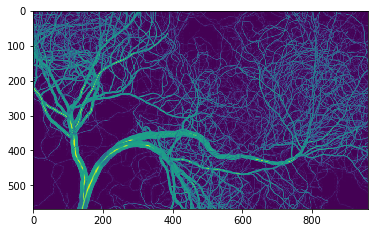Any Op that requires a RandomAccessibleInterval can run on a numpy array that has been passed to to_java. Remember that this method creates a view, meaning that the Op is modifying the underlying Python object:

Let's run a Difference of Gaussians on our numpy image using ImageJ:

In :
result = np.zeros(img.shape)
# these sigmas will be nice for the larger sections
sigma1 = 8
sigma2 = 2
# note the use of to_java on img and result to turn the numpy images into RAIs
ij.op().filter().dog(
ij.py.to_java(result),
ij.py.to_java(img),
sigma1,
sigma2)
# purple highlights the edges of the vessels, green highlights the centers
ij.py.show(result, cmap = 'PRGn')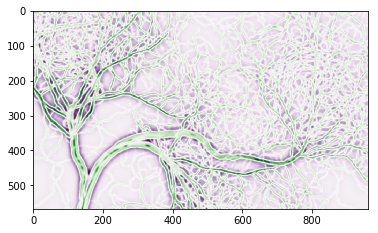## Send an ImageJ image into NumPy: ij.py.from_java¶

from_java works in reverse of to_java and can be used to further process ImageJ data types with numpy, scikit-image, etc.

Open an image from the url using the IJ scripting interface and then send it to a numpy array.

In :
url_colony = 'https://wsr.imagej.net/images/Cell_Colony.jpg'

cell_colony = ij.io().open(url_colony)

# Send it to numpy
numpy_colony = ij.py.from_java(cell_colony)

# Display the image
ij.py.show(numpy_colony, cmap='gray')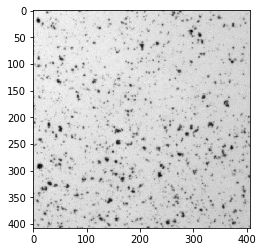### Warning: RGB and other axis conventions must be handled manually¶

Numpy is reverse indexed from ImageJ, e.g. axis order in numpy is ZYX and in ImageJ is (by default) XYZ. In addition, numpy and matplotlib have alimited understanding of axis conventions and only natively handle 3-channel RGB images as YXC. However, conversion between numpy and ImageJ is currently handled by simply reversing the axis order, so taking an ImageJ RGB -> numpy needs an additional step to plot correctly.

A future update will add ways of handling this using pyimagej, but this is currently in the hands of the user. For the time being this is how you can get around the issue:

In :
# load the image into IJ
ij_img = ij.io().open('https://samples.fiji.sc/new-lenna.jpg')

# Convert the image to a numpy array
img_from_ij = ij.py.from_java(ij_img)

np.shape(img_from_ij)

Out:
(3, 1279, 853)

Note that the channel dimension comes first. Now that we have the image we can ready it for plotting:

In :
# Hint: There are two barriers to plotting the image: the axis order, and that matplotlib only plot 8-bit RGB images
# Convert to 8-bit
img_as_8bit = img_from_ij.astype(int)

# Fix the axis order
img_as_rgb = np.moveaxis(img_as_8bit, 0, -1)

# Plot the image
ij.py.show(img_as_rgb)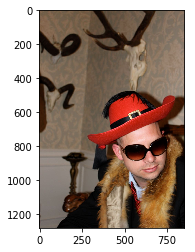## Convenience methods of ij.py¶

These methods can be helpful, especially if you do not know beforehand of which type your image is.

### ij.py.dims¶

This can be used to determine the dimensions of a numpy or ImageJ image:

In :
# numpy image
img1 = np.zeros([10, 10])
print(ij.py.dims(img1))

# imagej image
img2 = ij.py.to_java(img1)
print(ij.py.dims(img2))

(10, 10)
[10, 10]


### ij.py.new_numpy_image¶

Takes a single image argument, which can either be a numpy image or an imagej image

In :
# create a new numpy image from a numpy image
img3 = ij.py.new_numpy_image(img1)
print(type(img3))

# create a new numpy image from an imagej image
img4 = ij.py.new_numpy_image(img2)
print(type(img4))

<class 'numpy.ndarray'>
<class 'numpy.ndarray'>


## Running macros, scripts, and plugins¶

### Using ImageJ macros: ij.py.run_macro¶

Running an IJ1 style macro is as simple as providing the macro code in a string, and the arguments in a dictionary to run_macro. Modify the following cell to print your name, age, and city.

In :
macro = """
#@ String name
#@ int age
#@ String city
#@output Object greeting
greeting = "Hello " + name + ". You are " + age + " years old, and live in " + city + "."
"""
args = {
'name': 'Chuckles',
'age': 13,
'city': 'Nowhere'
}
result = ij.py.run_macro(macro, args)
print(result.getOutput('greeting'))

Hello Chuckles. You are 13 years old, and live in Nowhere.


Running scripts in other languages is similar, but you also have to specify the file extension for the scripting language it is written in.

In :
language_extension = 'ijm'
result_script = ij.py.run_script(language_extension, macro, args)
print(result_script.getOutput('greeting'))

Hello Chuckles. You are 13 years old, and live in Nowhere.


### Example: Run a plugin: ij.py.run_plugin¶

Finally, running plugins works in the same manner as macros. You simply enter the plugin name as a string and the arguments in a dict. For the few plugins that use IJ2 style macros (i.e., explicit booleans in the recorder), set the optional variable ij1_style=False

This example works with IJ1 windows, opening images entirely within IJ and then getting the results. Working with IJ1 windows requires importing another class, which is done using the jnius framework. The jnius.autoclass function can import other java classes for similar purposes.

In :
from jnius import autoclass
WindowManager = autoclass('ij.WindowManager')
ij.py.run_macro("""run("Blobs (25K)");""")
blobs = WindowManager.getCurrentImage()
print(blobs)

<ij.ImagePlus at 0x141ee2780 jclass=ij/ImagePlus jself=<LocalRef obj=0x7fbe9bf17f88 at 0x142a26470>>

In :
ij.py.show(blobs)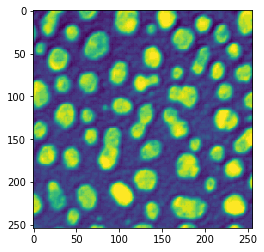We can now run plugins that require open IJ1 windows on blobs

In :
plugin = 'Mean'
args = {
}

ij.py.run_plugin(plugin, args)

Out:
<org.scijava.script.ScriptModule at 0x144dc62b0 jclass=org/scijava/script/ScriptModule jself=<LocalRef obj=0x7fbe9be36770 at 0x141eeb0f0>>
In :
result = WindowManager.getCurrentImage()
result = ij.py.show(result)You can list any active IJ1 windows with the following command.

In :
print(ij.py.from_java(ij.window().getOpenWindows()))

['blobs.gif']


You can close any IJ1 windows through the following command.

In :
ij.window().clear()
print(ij.py.from_java(ij.window().getOpenWindows()))

[]


## Visualizing large images¶

Before we begin: how much memory is Java using right now?

In :
from jnius import autoclass
Runtime = autoclass('java.lang.Runtime')
def java_mem():
rt = Runtime.getRuntime()
mem_max = rt.maxMemory()
mem_used = rt.totalMemory() - rt.freeMemory()
return '{} of {} MB ({}%)'.format(
int(mem_used / 2**20),
int(mem_max / 2**20),
int(100 * mem_used / mem_max))
java_mem()

Out:
'53 of 3641 MB (1%)'

Now let's open an obnoxiously huge synthetic dataset:

In :
big_data = ij.scifio().datasetIO().open('lotsofplanes&lengths=512,512,16,1000,10000&axes=X,Y,Channel,Z,Time.fake')


How many total samples does this image have?

In :
import numpy as np
dims = [big_data.dimension(d) for d in range(big_data.numDimensions())]
pix = np.prod(dims)
str(pix / 2**40) + " terapixels"

Out:
'38.14697265625 terapixels'

And how much did memory usage in Java increase?

In :
java_mem()

Out:
'823 of 3641 MB (22%)'

Let's visualize this beast. First, we define a function for slicing out a single plane:

In :
def plane(image, pos):
while image.numDimensions() > 2:
image = ij.op().transform().hyperSliceView(image, image.numDimensions() - 1, pos[-1])
pos.pop()
return ij.py.from_java(image)

ij.py.show(plane(big_data, [0, 0, 0]))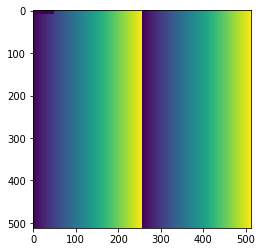But we can do better. Let's provide some interaction. First, a function to extract the non-planar axes as a dict:

In :
from jnius import autoclass, cast
CalibratedAxis = autoclass('net.imagej.axis.CalibratedAxis')

def axes(dataset):
axes = {}
for d in range(2, dataset.numDimensions()):
axis = cast(CalibratedAxis, dataset.axis(d))
label = axis.type().getLabel()
length = dataset.dimension(d)
axes[label] = length
return axes

axes(big_data)

Out:
{'Channel': 16, 'Z': 1000, 'Time': 10000}

And now, we have the tools we need to use ipywidgets.interact for any N-dimensional image!

In :
import ipywidgets, matplotlib

widgets = {}
for label, length in axes(big_data).items():
widgets[label] = ipywidgets.IntSlider(description=label, max=length-1)

def f(**kwargs):
matplotlib.pyplot.imshow(plane(big_data, list(kwargs.values())), cmap='gray')
ipywidgets.interact(f, **widgets);


## Troubleshooting¶

### I can't pass my numpy image through to an Op¶

ij.py is really good at converting numpy images into RandomAccessibleIntervals. However many Ops, like addPoissonNoise, take other forms of ImageJ images, like IterableInterval.

In :
print(ij.op().help('filter.addPoissonNoise'))

Available operations:
(RealType out) =
RealType out,
RealType in,
long seed?)
(IterableInterval out) =

We can't call this Op on a numpy array since it is a specialized type of RandomAccessibleInterval, which does not extend IterableInterval.
# Create a numpy image using scikit